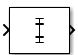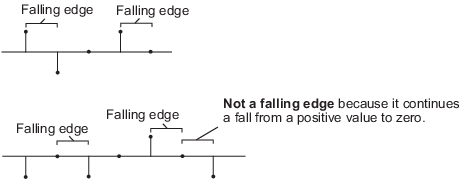# Standard Deviation

Standard deviation of input or sequence of inputs

•Libraries:
DSP System Toolbox / Statistics

## Description

The Standard Deviation block computes the standard deviation of each row or column of the input, or along vectors of a specified dimension of the input. It can also compute the standard deviation of the entire input. You can specify the dimension using the Find the standard deviation value over parameter. The Standard Deviation block can also track the standard deviation in a sequence of inputs over a period of time. To track the standard deviation in a sequence of inputs, select the Running standard deviation parameter.

Note

The Running mode in the Standard Deviation block will be removed in a future release. To compute the running standard deviation in Simulink®, use the Moving Standard Deviation block instead.

## Ports

### Input

expand all

The block accepts real-valued or complex-valued multichannel and multidimensional inputs.

This port is unnamed until you select the Running standard deviation parameter and set the Reset port parameter to any option other than `None`.

Data Types: `single` | `double`
Complex Number Support: Yes

Specify the reset event that causes the block to reset the running standard deviation. The sample time of the Rst input must be a positive integer multiple of the input sample time.

#### Dependencies

To enable this port, select the Running standard deviation parameter and set the Reset port parameter to any option other than `None`.

Data Types: `single` | `double` | `int8` | `int16` | `int32` | `uint8` | `uint16` | `uint32` | `Boolean`

### Output

expand all

The data type of the output matches the data type of the input.

When you do not select the Running standard deviation parameter, the block computes the standard deviation in each row or column of the input, or along vectors of a specified dimension of the input. It can also compute the standard deviation of the entire input at each individual sample time. Each element in the output array `y` is the standard deviation of the corresponding column, row, or entire input. The output array `y` depends on the setting of the Find the standard deviation value over parameter. Consider a three-dimensional input signal of size M-by-N-by-P. When you set Find the standard deviation value over to:

• `Entire input` — The output at each sample time is a scalar that contains the standard deviation of the M-by-N-by-P input matrix.

• `Each row` — The output at each sample time consists of an M-by-1-by-P array, where each element contains the standard deviation of each vector over the second dimension of the input. For an M-by-N matrix input, the output at each sample time is an M-by-1 column vector.

• `Each column` — The output at each sample time consists of a 1-by-N-by-P array, where each element contains the standard deviation of each vector over the first dimension of the input. For an M-by-N matrix input, the output at each sample time is a 1-by-N row vector.

In this mode, the block treats length-M unoriented vector inputs as M-by-1 column vectors.

• `Specified dimension` — The output at each sample time depends on the value of the Dimension parameter. If you set the Dimension to `1`, the output is the same as when you select ```Each column```. If you set the Dimension to `2`, the output is the same as when you select ```Each row```. If you set the Dimension to `3`, the output at each sample time is an M-by-N matrix containing the standard deviation of each vector over the third dimension of the input.

When you select Running standard deviation, the block tracks the standard deviation of each channel in a time sequence of inputs. In this mode, you must also specify a value for the Input processing parameter.

• `Elements as channels (sample based)` — The block treats each element of the input as a separate channel. For a three-dimensional input signal of size M-by-N-by-P, the block outputs an M-by-N-by-P array. Each element yijk of the output contains the standard deviation of the element uijk for all inputs since the last reset.

When a reset event occurs, the running standard deviation yijk in the current frame is reset to the element uijk.

• `Columns as channels (frame based)` — The block treats each column of the input as a separate channel. This option does not support input signals with more than two dimensions. For a two-dimensional input signal of size M-by-N, the block outputs an M-by-N matrix. Each element yij of the output contains the standard deviation of the elements in the jth column of all inputs since the last reset, up to and including the element uij of the current input.

When a reset event occurs, the running standard deviation for each channel becomes the standard deviation of all the samples in the current input frame, up to and including the current input sample.

Data Types: `single` | `double`

## Parameters

expand all

### Main Tab

When you select the Running standard deviation parameter, the block tracks the standard deviation value of each channel in a time sequence of inputs.

• `Each column` — The block outputs the standard deviation over each column.

• `Each row` — The block outputs the standard deviation over each row.

• `Entire input` — The block outputs the standard deviation over the entire input.

• `Specified dimension` — The block outputs the standard deviation over the dimension, specified in the Dimension parameter.

#### Dependencies

To enable this parameter, clear the Running standard deviation parameter.

Specify the dimension (one-based value) of the input signal over which the standard deviation is computed. The value of this parameter must be greater than 0 and less than the number of dimensions in the input signal.

#### Dependencies

To enable this parameter, set Find the standard deviation value over to ```Specified dimension```.

• `Columns as channels (frame based)` — The block treats each column of the input as a separate channel. This option does not support input signals with more than two dimensions. For a two-dimensional input signal of size M-by-N, the block outputs an M-by-N matrix. Each element yij of the output contains the standard deviation of the elements in the jth column of all inputs since the last reset, up to and including the element uij of the current input.

When a reset event occurs, the running standard deviation for each channel becomes the standard deviation of all the samples in the current input frame, up to and including the current input sample.

• `Elements as channels (sample based)` — The block treats each element of the input as a separate channel. For a three-dimensional input signal of size M-by-N-by-P, the block outputs an M-by-N-by-P array. Each element yijk of the output contains the standard deviation of the element uijk for all inputs since the last reset.

When a reset event occurs, the running standard deviation yijk in the current frame is reset to the element uijk.

Variable-Size Inputs

When your inputs are of variable size, and you select the Running standard deviation parameter, then:

• If you set the Input processing parameter to ```Elements as channels (sample based)```, the state is reset.

• If you set the Input processing parameter to ```Columns as channels (frame based)```, then:

• When the input size difference is in the number of channels (number of columns), the state is reset.

• When the input size difference is in the length of channels (number of rows), no reset occurs and the running operation is carried out as usual.

#### Dependencies

To enable this parameter, select the Running standard deviation parameter.

The block resets the running standard deviation whenever a reset event is detected at the optional Rst port. The reset sample time must be a positive integer multiple of the input sample time.

When a reset event occurs while the Input processing parameter is set to ```Elements as channels (sample based)```, the running standard deviation for each channel is initialized to the value in the corresponding channel of the current input. Similarly, when the Input processing parameter is set to `Columns as channels (frame based)`, the running standard deviation for each channel becomes the standard deviation of all the samples in the current input frame, up to and including the current input sample.

Use this parameter to specify the reset event.

• `None` — Disables the Rst port.

• `Rising edge` — Triggers a reset operation when the Rst input does one of the following:

• Rises from a negative value to either a positive value or zero.

• Rises from zero to a positive value, where the rise is not a continuation of a rise from a negative value to zero.• `Falling edge` — Triggers a reset operation when the Rst input does one of the following:

• Falls from a positive value to a negative value or zero.

• Falls from zero to a negative value, where the fall is not a continuation of a fall from a positive value to zero.• `Either edge` — Triggers a reset operation when the Rst input is a `Rising edge` or `Falling edge`.

• `Non-zero sample` — Triggers a reset operation at each sample time, when the Rst input is not zero.

Note

When running simulations in the Simulink multitasking mode, reset signals have a one-sample latency. Therefore, when the block detects a reset event, there is a one-sample delay at the reset port rate before the block applies the reset. For more information on latency and the Simulink tasking modes, see Excess Algorithmic Delay (Tasking Latency) and Time-Based Scheduling and Code Generation (Simulink Coder).

#### Dependencies

To enable this parameter, select the Running standard deviation parameter.

## Block Characteristics

 Data Types `double` | `single` Direct Feedthrough `no` Multidimensional Signals `no` Variable-Size Signals `yes` Zero-Crossing Detection `no`

expand all

expand all

## Version History

Introduced before R2006a